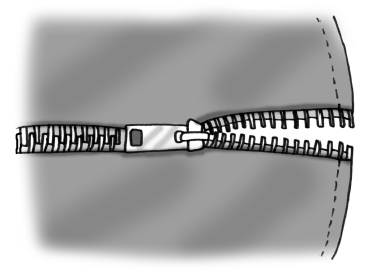### Home > A2C > Chapter 12 > Lesson 12.1.3 > Problem12-51

12-51.

Lorna is looking at the series $5+3+10+8+15+\dots+400+398$.The sequence definitely isn't arithmetic, but it does have a pattern,” Lorna told her team, “I wonder if we can still find the sum of the series?”

1. What patterns do you see in Lorna's series? Describe them as completely as you can.

Look at the first four terms. There are repetitive decreases of $2$ and increases of $7$.

2. Do you agree with Lorna's statement that this sequence is not arithmetic? Justify your conclusion. Do you think it is still possible to find a sum for the series?

Can you write an equation for the terms of the sequence in the form $t(n)=a+b(n-1)?$

3. I think I see it!” Lorna exclaimed. “I think we can separate the terms into the two different arithmetic series shown below, kind of like unzipping a zipper.” Her work is shown below.
$5+10+15+\dots+395+400$
$3+8+13+\dots+393+398$

How can you use Lorna's two series to find the sum of the original series? State the sum and describe your process.

Find the sum of these two new series independently, and add the two sums together to find the original sum.

$32{,}240$